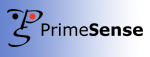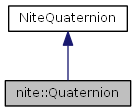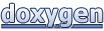# NiTE 2.0: nite::Quaternion Class Reference

#### NiTE 2.0NiTE 2.0
nite::Quaternion Class Reference

`#include <NiTE.h>`

Inheritance diagram for nite::Quaternion:[legend]

List of all members.

## Public Member Functions

Quaternion ()
Quaternion (float w, float x, float y, float z)

## Detailed Description

Represents a Quaternion. The Quaternion is stored as four floating point numbers. (The quaternions are a number system that extends the complex number system from two dimensions to four.)

## Constructor & Destructor Documentation

 nite::Quaternion::Quaternion ( ) ` [inline]`

Default Constructor. Creates a new Quaternion with the value of all four components set to zero.

 nite::Quaternion::Quaternion ( float w, float x, float y, float z ) ` [inline]`

Constructor. Creates a new Quaternion with each component specified.

Parameters:
 [in] w Desired first quaternion component value. [in] x Desired second quaternion component value. [in] y Desired third quaternion component value. [in] z Desired fourth quaternion component value.

The documentation for this class was generated from the following file:
Generated on Thu Jun 6 2013 17:48:15 for NiTE 2.0 by1.7.5.1